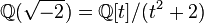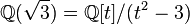Quiz:Splitting field

Rational and ambivalent

An ambivalent group is a group in which every element is conjugate to its inverse. For a finite group, this is equivalent to every character in characteristic zero being real-valued.

A rational group is a group in which any two elements generating the same cyclic subgroup are conjugate. For a finite group, this is equivalent to every character in characteristic zero being rational-valued.

1 There are only finitely many values$n$ such that the alternating group of degree$n$ is ambivalent. For which of the following values of$n$ is the alternating group$A_n$ of degree$n$ not ambivalent?

 5 6 8 9 10

2 What is the smallest possible order of an ambivalent group that is not a rational group?

 6 8 10 12 16

3 Which of the following conditions on a natural number$n > 1$ is necessary and sufficient for there to be an ambivalent group of order$n$?$n$ must be a prime power$n$ must be divisible by at least two primes$n$ must be even$n$ must be divisible by one of the primes 2, 3, or 5$n$ must be divisible by the square of a prime

4 A rational-representation group is a group all of whose irreducible representations in characteristic zero can be realized over the rationals, i.e., the rational numbers are a splitting field for the group. All the groups below are rational groups. Which of them is not a rational-representation group?

 symmetric group:S3 dihedral group:D8 (see linear representation theory of dihedral group:D8) quaternion group (see linear representation theory of quaternion group) direct product of D8 and Z2 symmetric group:S4

Splitting fields in characteristic zero

1 For a group of order 27, what is the maximum possible degree of a minimal splitting field in characteristic zero as an extension over the rationals?

 3 6 9 18 27

2 Of the five groups of order 8 (see also linear representation theory of groups of order 8), there is exactly one group that does not have a unique minimal splitting field in characteristic zero, i.e., there are multiple non-isomorphic minimal splitting fields in characteristic zero. Which group is this?

 cyclic group:Z8 direct product of Z4 and Z2 dihedral group:D8 quaternion group elementary abelian group:E8

3 It's obvious that for a finite group, if the field generated by character values in characteristic zero is a splitting field, then it is the unique minimal splitting field. Further, it is true that if every irreducible representation has Schur index one, then the field generated by character values is a minimal splitting field. It may, however, be possible that the field generated by character values is a splitting field, despite the existence of irreducible representations with Schur index more than one. Which of the following groups is an example of this phenomenon?

 dihedral group:D8 (order 8, see linear representation theory of dihedral group:D8) quaternion group (order 8, see linear representation theory of quaternion group) dihedral group:D16 (order 16, see linear representation theory of dihedral group:D16) semidihedral group:SD16 (order 16, see linear representation theory of semidihedral group:SD16) special linear group:SL(2,3) (order 24, see linear representation theory of special linear group:SL(2,3))

4 Which of the fields does not occur as the field generated by character values for a finite group of order 16? See also linear representation theory of groups of order 16#Splitting field.$\mathbb{Q}$ -- the field of rational numbers$\mathbb{Q}(i) = \mathbb{Q}[t]/(t^2 + 1)$$\mathbb{Q}(\sqrt{2}) = \mathbb{Q}[t]/(t^2 - 2)$$\mathbb{Q}(\sqrt{-2}) = \mathbb{Q}[t]/(t^2 + 2)$$\mathbb{Q}(\sqrt{3}) = \mathbb{Q}[t]/(t^2 - 3)$

Rings of realization

1 Consider the following integral domains$R$ of characteristic zero. For which of them is it not true that any linear representation realized over the field of fractions of$R$ can also, based on a suitable choice of basis, be realized with all matrix entries coming from$R$?$\mathbb{Z}$ -- the ring of integers$\mathbb{Z}[i] = \mathbb{Z}[t]/(t^2 + 1)$ -- the ring of Gaussian integers$\mathbb{Z}[\sqrt{5}] = \mathbb{Z}[t]/(t^2 - 5)$$\mathbb{Z}[(1 + \sqrt{5})/2]$$\mathbb{Z}[t]/(t^2 + t + 1)$